## An organ pipe open at both ends has two successive harmonics with frequencies of 220 Hz and 240 Hz. What is the length of the pipe? The spee

Question

An organ pipe open at both ends has two successive harmonics with frequencies of 220 Hz and 240 Hz. What is the length of the pipe? The speed of sound is 343 m/s in air.

in progress 0
5 months 2021-08-05T01:03:18+00:00 1 Answers 12 views 0

## Answers ( )

The  value is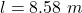Explanation:

From the question we are told that

The  frequencies of two successive harmonics  is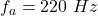,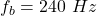The  speed of sound in the air is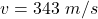Generally a harmonic frequency is mathematically represented as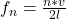here l is the length of the pipe

n is the order of position of the harmonics

Now since we do not know the order of the given harmonic frequencies but we are told that they are successive then the frequencies can be mathematically represented as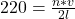and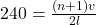So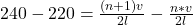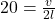=>